# AIR NAVIGATION Part 2 The Triangle of Velocities

• Slides: 41AIR NAVIGATION Part 2 The Triangle of Velocities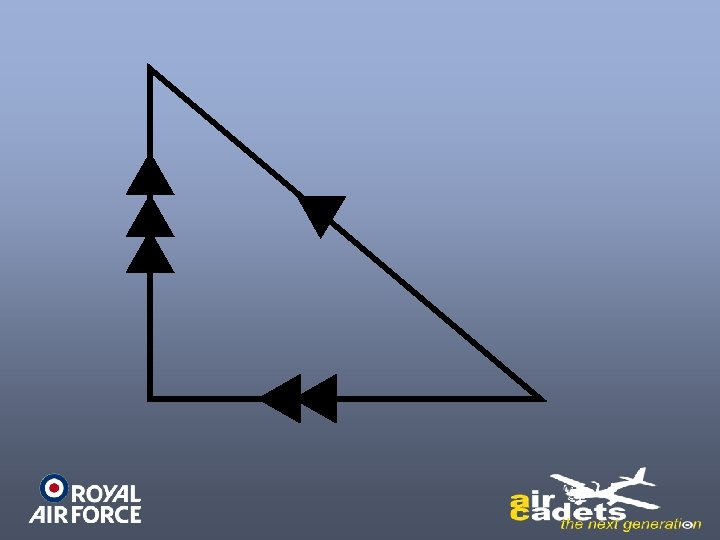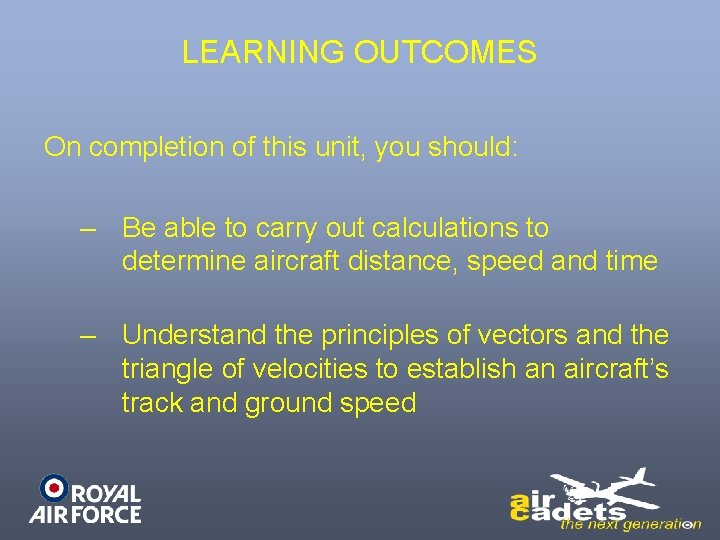LEARNING OUTCOMES On completion of this unit, you should: – Be able to carry out calculations to determine aircraft distance, speed and time – Understand the principles of vectors and the triangle of velocities to establish an aircraft’s track and ground speedLEARNING OUTCOMES – Understand the principles of the 1 in 60 rule – Understand the types of compass systems used for air navigation, how they work and their limitations – Know the hazards that weather presents to aviationThe Triangle of Velocities • It is now necessary to consider the wind, when we talk about flying, which is simply air that is moving.Vectors & Velocity • Whenever we talk about aircraft or wind movement, we must always give both the direction and speed of the movement. • Direction and speed together are called a velocity.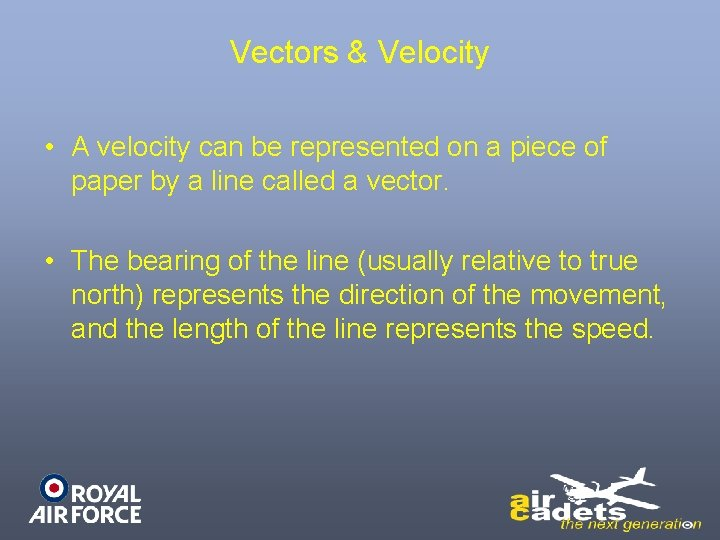Vectors & Velocity • A velocity can be represented on a piece of paper by a line called a vector. • The bearing of the line (usually relative to true north) represents the direction of the movement, and the length of the line represents the speed.True North Track of 070° Speed 120 kts (070/120) Track of 115° Speed 240 kts (115/240)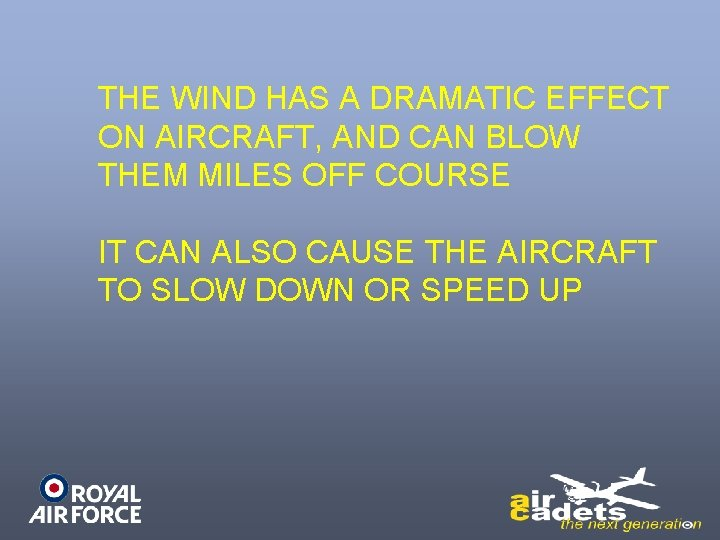THE WIND HAS A DRAMATIC EFFECT ON AIRCRAFT, AND CAN BLOW THEM MILES OFF COURSE IT CAN ALSO CAUSE THE AIRCRAFT TO SLOW DOWN OR SPEED UP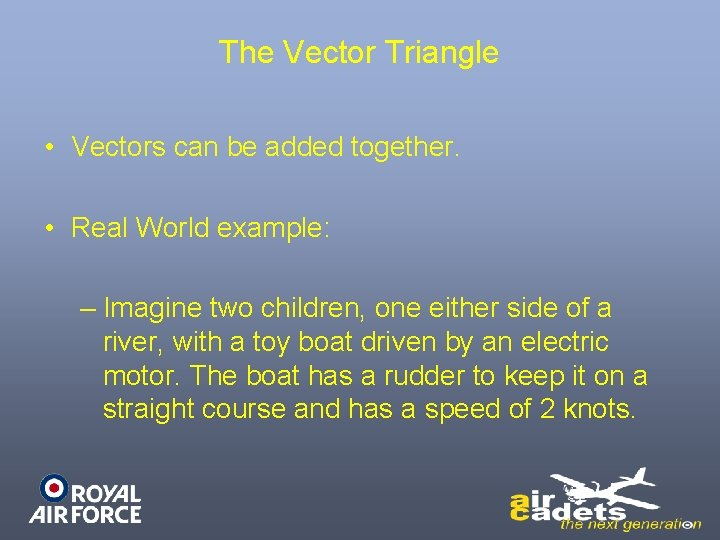The Vector Triangle • Vectors can be added together. • Real World example: – Imagine two children, one either side of a river, with a toy boat driven by an electric motor. The boat has a rudder to keep it on a straight course and has a speed of 2 knots.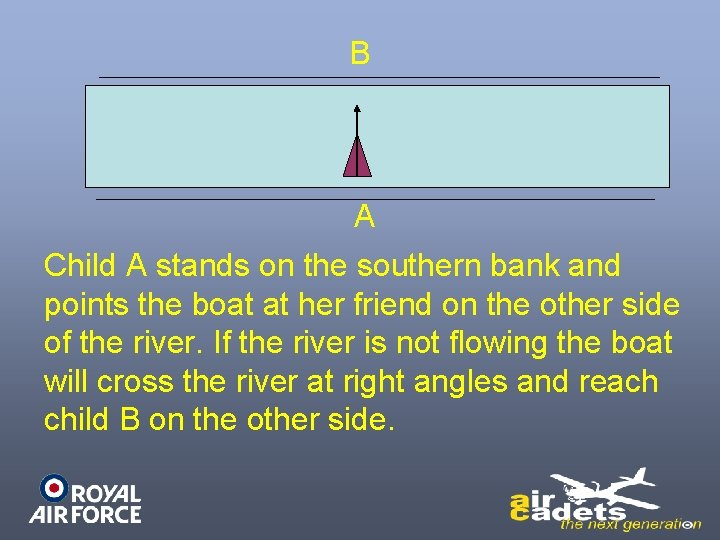B A Child A stands on the southern bank and points the boat at her friend on the other side of the river. If the river is not flowing the boat will cross the river at right angles and reach child B on the other side.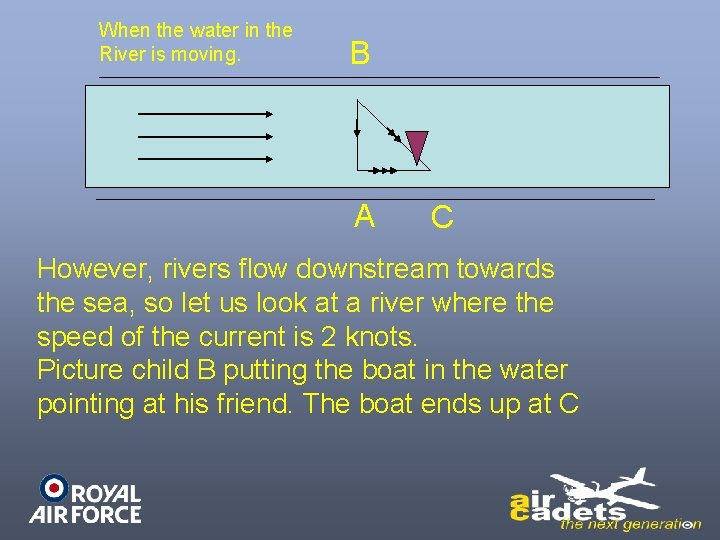When the water in the River is moving. B A C However, rivers flow downstream towards the sea, so let us look at a river where the speed of the current is 2 knots. Picture child B putting the boat in the water pointing at his friend. The boat ends up at C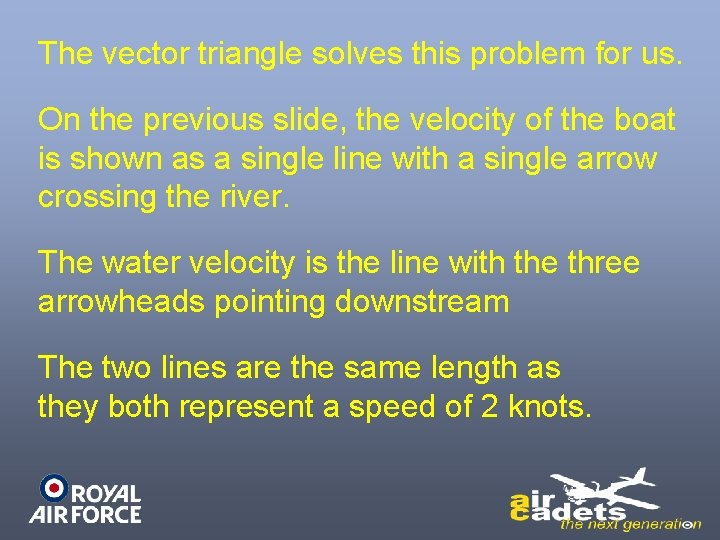The vector triangle solves this problem for us. On the previous slide, the velocity of the boat is shown as a single line with a single arrow crossing the river. The water velocity is the line with the three arrowheads pointing downstream The two lines are the same length as they both represent a speed of 2 knots.You then join the two ends and make a triangle. The third side of the vector triangle (called the resultant and indicated by arrowheads) represents the actual movement of the boat as it crabs across the river. By use of Pythagoras’s theorem it can be shown that the speed of the boat over the riverbed is 2. 83 knots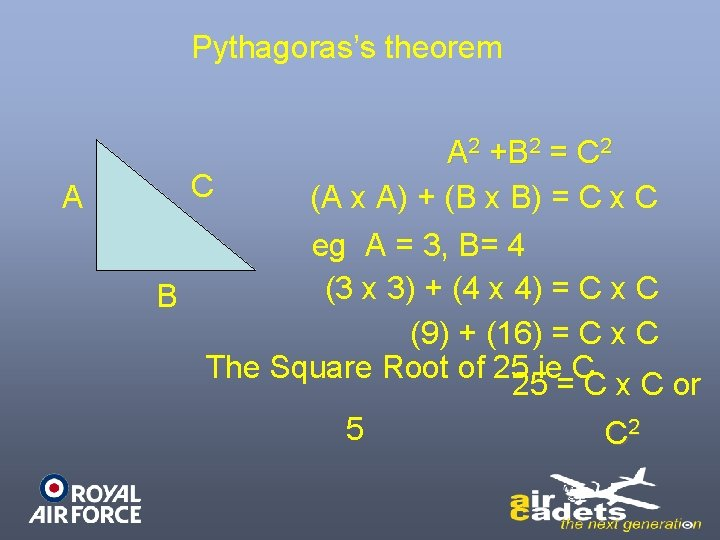Pythagoras’s theorem A A 2 +B 2 = C 2 C (A x A) + (B x B) = C x C eg A = 3, B= 4 (3 x 3) + (4 x 4) = C x C B (9) + (16) = C x C The Square Root of 25 ie C 25 = C x C or 5 C 2The Air Triangle When the water in the River is moving. B A C Exactly the same triangle can be used to show the motion of a aircraft hrough the air which is itself moving.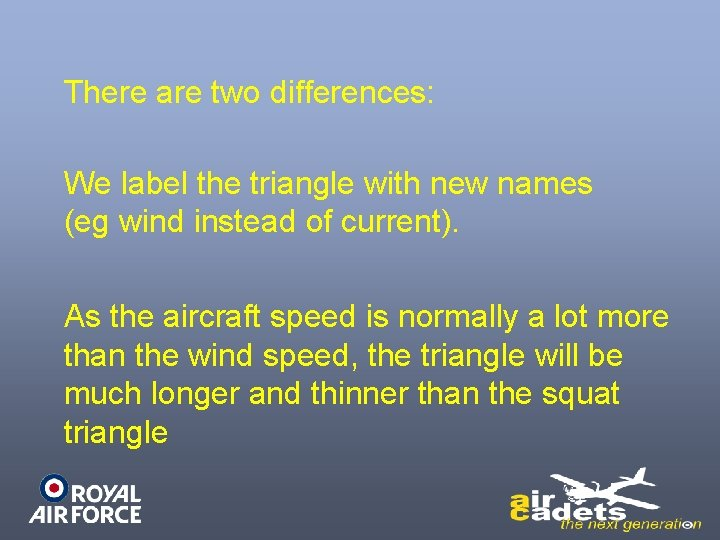There are two differences: We label the triangle with new names (eg wind instead of current). As the aircraft speed is normally a lot more than the wind speed, the triangle will be much longer and thinner than the squat triangle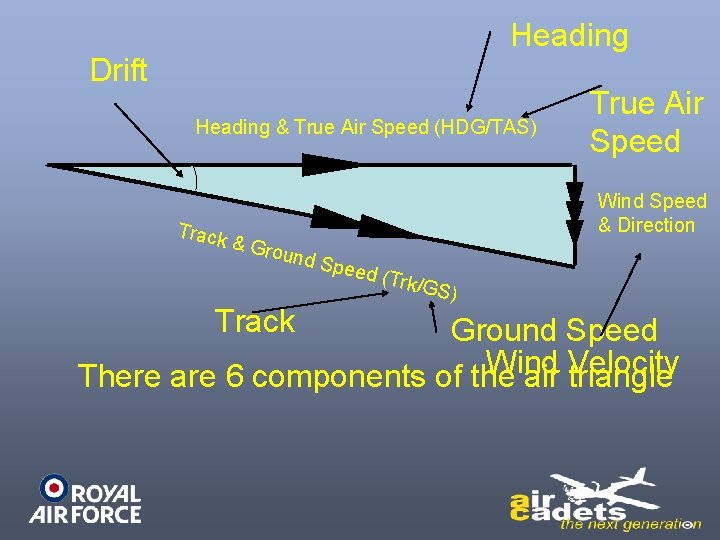Heading Drift Heading & True Air Speed (HDG/TAS) Track True Air Speed Wind Speed & Direction & Gro und S Track peed (Trk/G S) Ground Speed Wind There are 6 components of the air Velocity triangle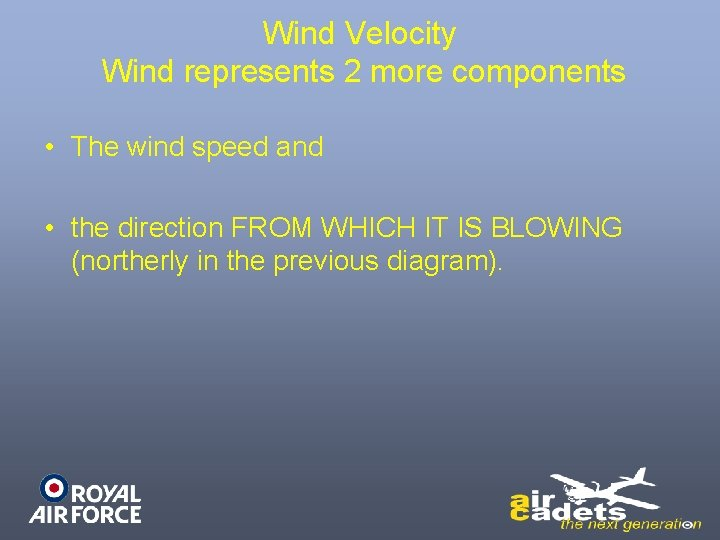Wind Velocity Wind represents 2 more components • The wind speed and • the direction FROM WHICH IT IS BLOWING (northerly in the previous diagram).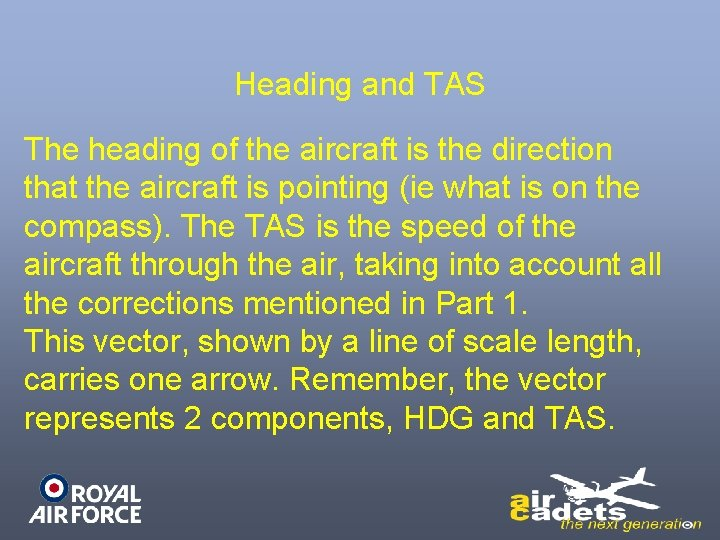Heading and TAS The heading of the aircraft is the direction that the aircraft is pointing (ie what is on the compass). The TAS is the speed of the aircraft through the air, taking into account all the corrections mentioned in Part 1. This vector, shown by a line of scale length, carries one arrow. Remember, the vector represents 2 components, HDG and TAS.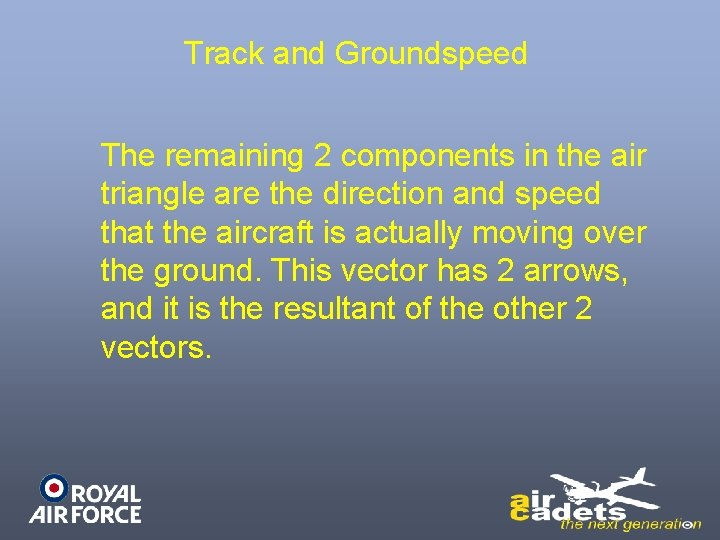Track and Groundspeed The remaining 2 components in the air triangle are the direction and speed that the aircraft is actually moving over the ground. This vector has 2 arrows, and it is the resultant of the other 2 vectors.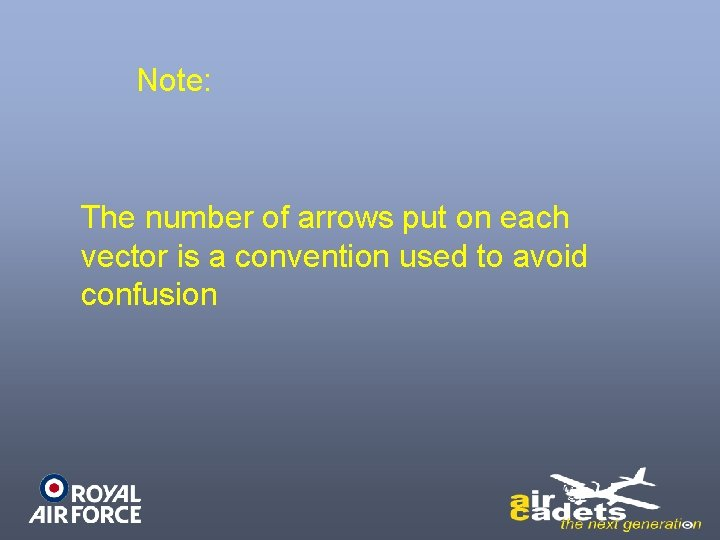Note: The number of arrows put on each vector is a convention used to avoid confusion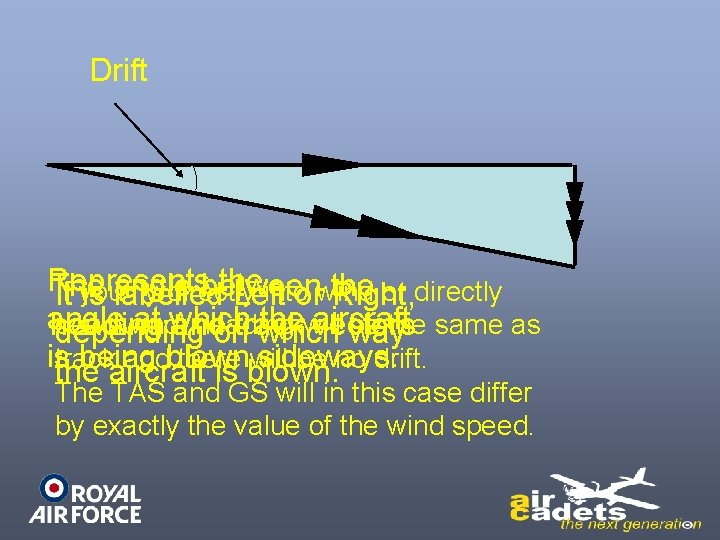Drift Represents the The angle between the If you fly directly intoorwind or directly It is labelled Left Right, angle at which thewill aircraft downwind, heading be the same as heading and vectors depending ontrack which way istrack being blown sideways. and there will be no drift. the aircraft is blown. The TAS and GS will in this case differ by exactly the value of the wind speed.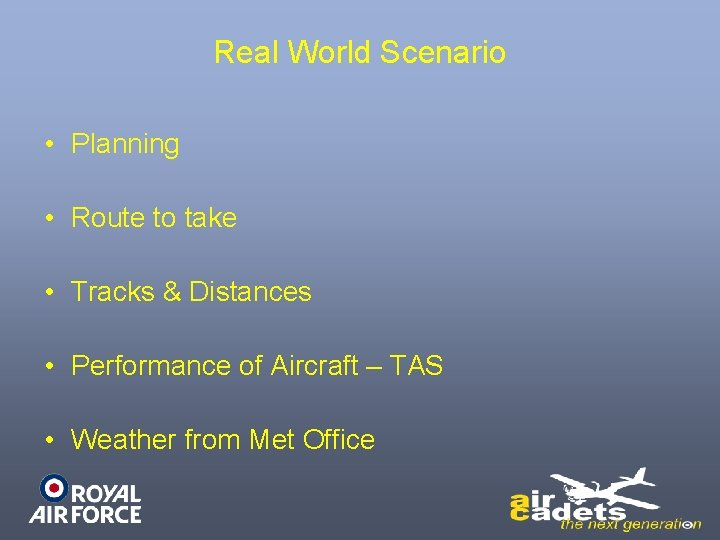Real World Scenario • Planning • Route to take • Tracks & Distances • Performance of Aircraft – TAS • Weather from Met OfficeReal World Scenario • We now identified the following: – TAS – TRK – Wind Speed & Direction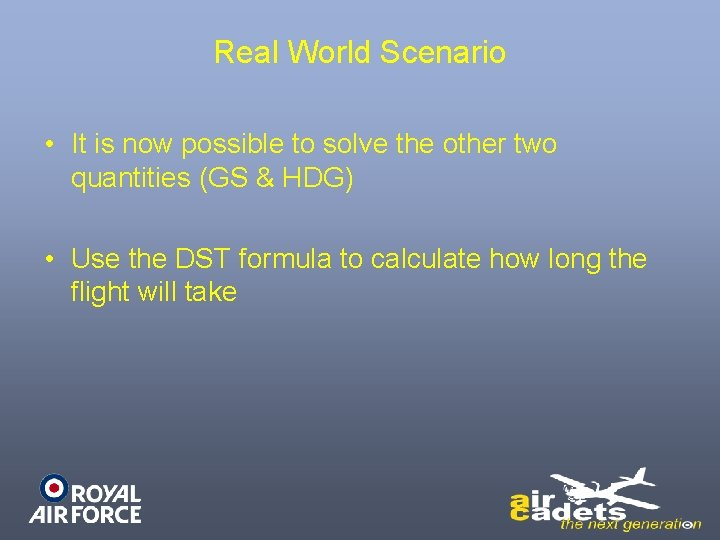Real World Scenario • It is now possible to solve the other two quantities (GS & HDG) • Use the DST formula to calculate how long the flight will takeReal World Scenario • The second scenario is in the air when we know the: – TAS and – HDGReal World Scenario • We can measure out TRK and GS by watching our changing position over the ground • From these 4 items, we can calculate the W/V.Real World Scenario • Finally, you know your heading and TAS and have a reliable W/V but are over a featureless area such as the sea and are unable to take any form of fix • By applying the W/V to your heading and TAS you can calculate your track and Groundspeed.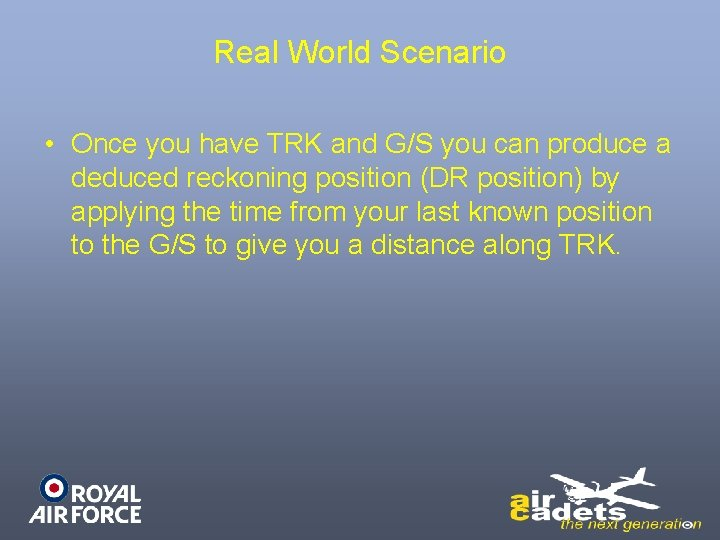Real World Scenario • Once you have TRK and G/S you can produce a deduced reckoning position (DR position) by applying the time from your last known position to the G/S to give you a distance along TRK.Computers • So far we have only talked about drawing vectors on paper • This is fine in the office or classroom but impossible in the cramped confines of a small aircraft.Dalton Computer • For many years, navigators have been using the Dalton hand held computer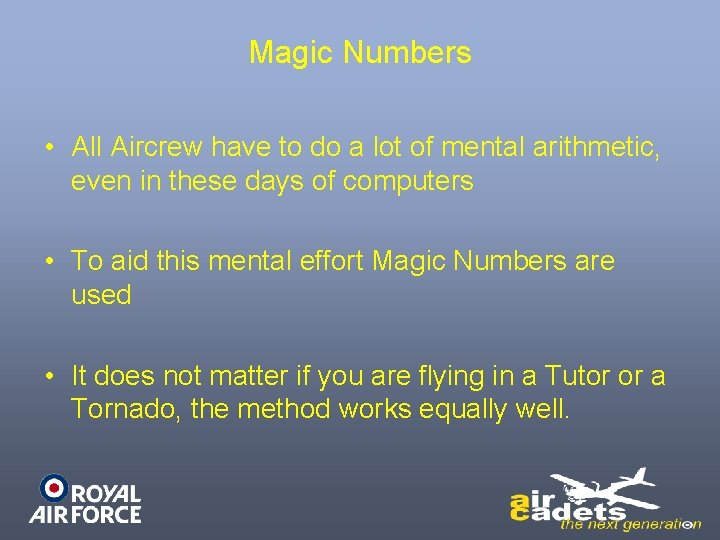Magic Numbers • All Aircrew have to do a lot of mental arithmetic, even in these days of computers • To aid this mental effort Magic Numbers are used • It does not matter if you are flying in a Tutor or a Tornado, the method works equally well.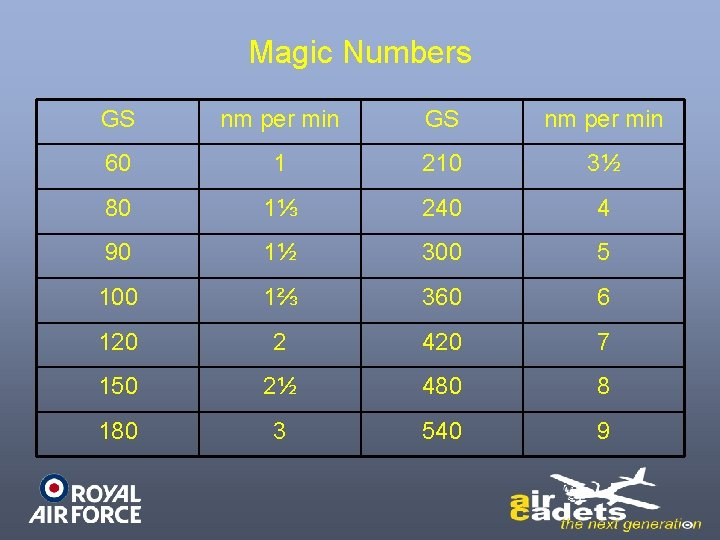Magic Numbers GS nm per min 60 1 210 3½ 80 1⅓ 240 4 90 1½ 300 5 100 1⅔ 360 6 120 2 420 7 150 2½ 480 8 180 3 540 9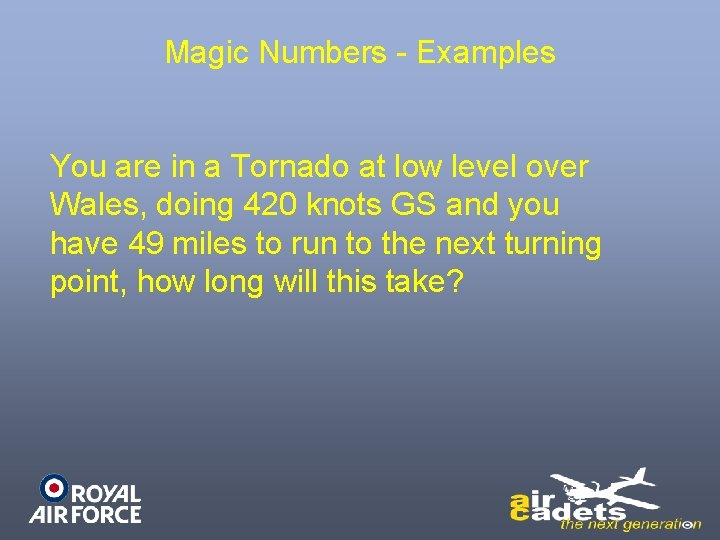Magic Numbers - Examples You are in a Tornado at low level over Wales, doing 420 knots GS and you have 49 miles to run to the next turning point, how long will this take?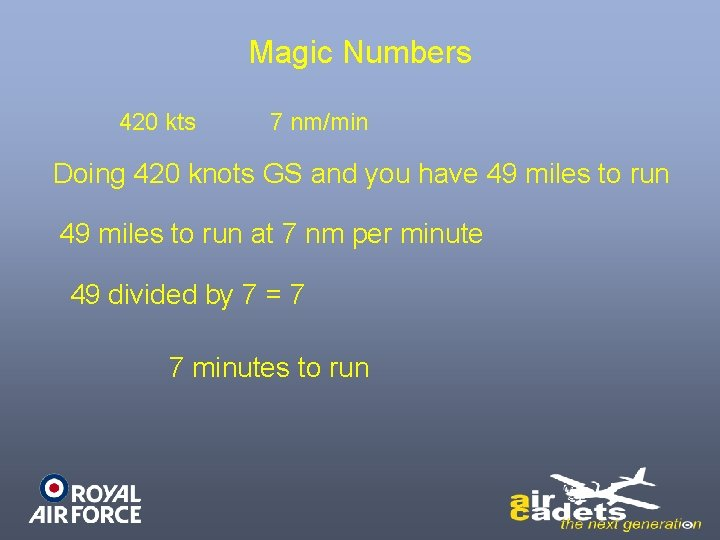Magic Numbers 420 kts 7 nm/min Doing 420 knots GS and you have 49 miles to run at 7 nm per minute 49 divided by 7 = 7 7 minutes to runAnother Example You are on a cross-country exercise in a Tutor, heading into wind at 80 knots 3 20 How 20 GS. long=will a 20 mile leg take? x 20 = 4 4 1⅓ 3 5 20 x 3 4 = 5 x 3 = 15 min 1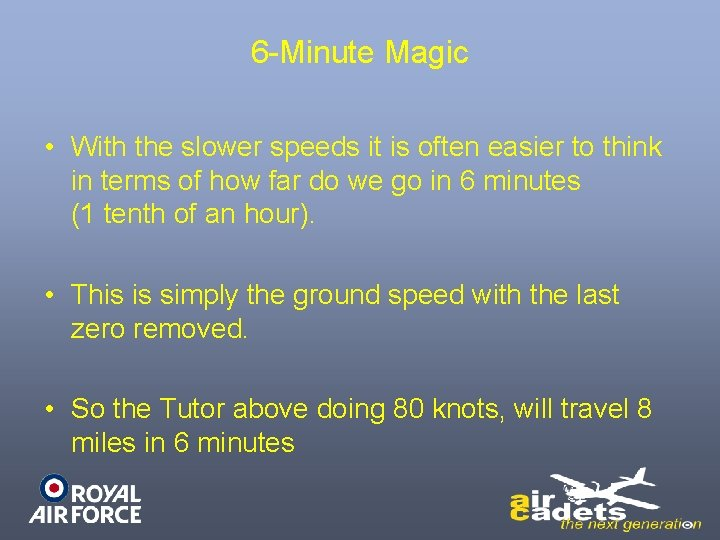6 -Minute Magic • With the slower speeds it is often easier to think in terms of how far do we go in 6 minutes (1 tenth of an hour). • This is simply the ground speed with the last zero removed. • So the Tutor above doing 80 knots, will travel 8 miles in 6 minutes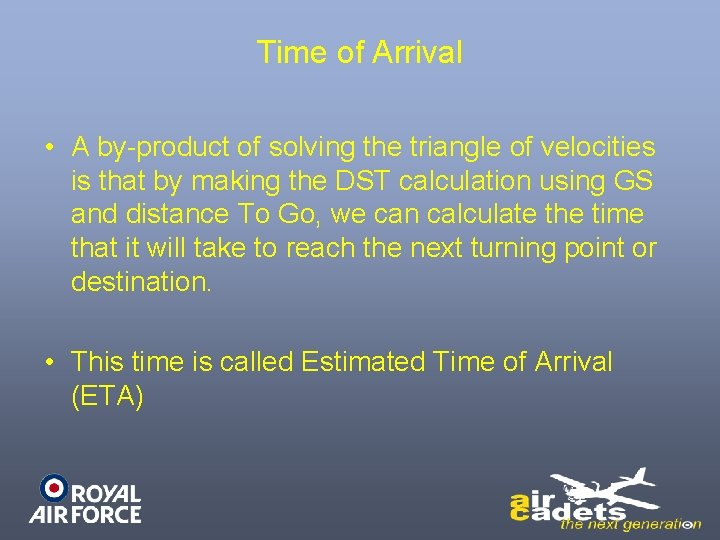Time of Arrival • A by-product of solving the triangle of velocities is that by making the DST calculation using GS and distance To Go, we can calculate the time that it will take to reach the next turning point or destination. • This time is called Estimated Time of Arrival (ETA)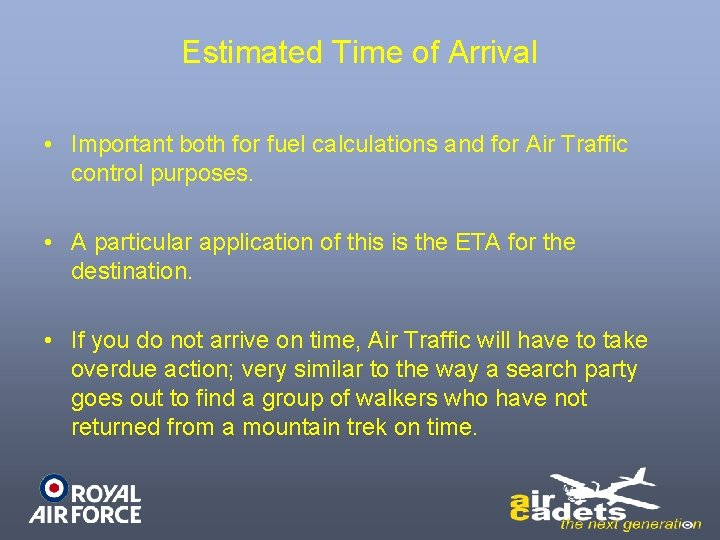Estimated Time of Arrival • Important both for fuel calculations and for Air Traffic control purposes. • A particular application of this is the ETA for the destination. • If you do not arrive on time, Air Traffic will have to take overdue action; very similar to the way a search party goes out to find a group of walkers who have not returned from a mountain trek on time.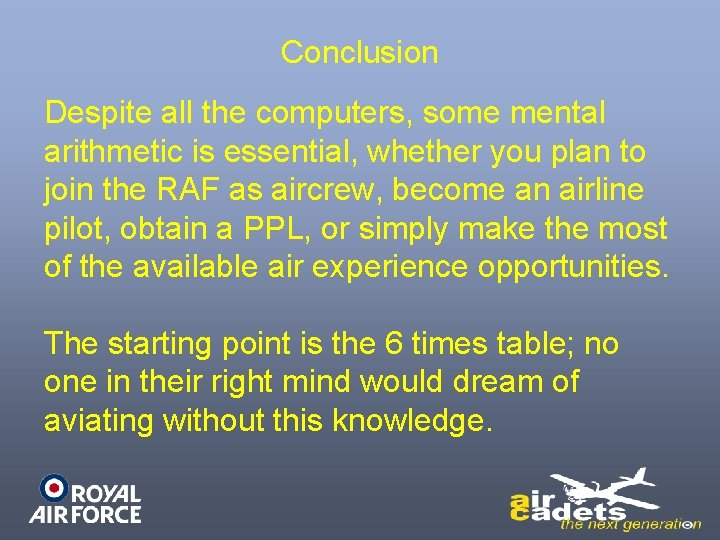Conclusion Despite all the computers, some mental arithmetic is essential, whether you plan to join the RAF as aircrew, become an airline pilot, obtain a PPL, or simply make the most of the available air experience opportunities. The starting point is the 6 times table; no one in their right mind would dream of aviating without this knowledge.